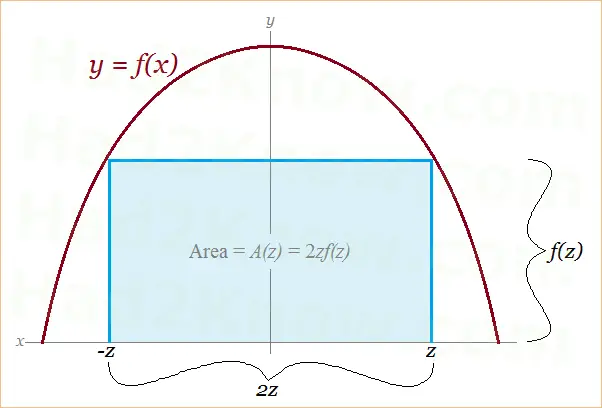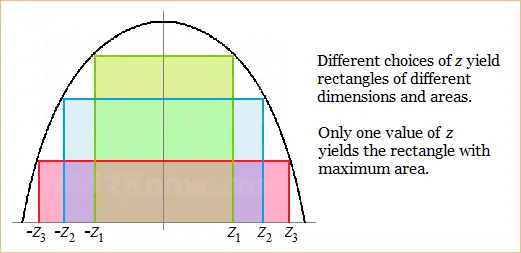# How to Find the Rectangle with Maximum Area Under a Curve

When you inscribe a rectangle beneath the curve of a symmetric function (such as a parabola, ellipse, catenary, or hyperbola), the rectangle's area is given by a new function. Using the techniques of calculus, you can take the derivative of this new function, set it equal to zero, and then solve for the critical points to find the dimensions of the largest rectangle.

To illustrate the method, first see the figure below:If you pick an arbitrary point (z, 0) on the x-axis along with its mirror image point (-z, 0), then you form the base of the rectangle whose width is 2z. Drawing a vertical line from (z, 0) to the point (z, f(z)), you generate the perpendicular side of the rectangle, whose height is f(z).

Thus, the function that gives area of the rectangle is A(z) = 2zf(z); you simply multiply the width and height. Using the product rule for differentiation, you find the derivative of this function is

A'(z) = 2f(z) + 2zf'(z).

When you set this equation equal to zero to find the critical point(s), you obtain

0 = 2f(z) + 2zf'(z)
-2f(z) = 2zf'(z)
-f(z) = zf'(z)

Solving this equation for z yields the critical point(s), one of which provides the dimensions for the rectangle of maximum area.### Example 1: Parabola

Suppose a parabola's equation is f(x) = 20 - 5x². This is the equation of a parabola whose y-intercept is (0, 20) and whose two x-intercepts are (2, 0) and (-2, 0).

The derivative of this function is f'(x) = -10x. Now plug these functions into the rectangle maximization equation above to find the critical point:

-f(z) = zf'(z)
-20 + 5z² = z(-10z)
-20 + 5z² = -10z²
-20 = -15z²
20 = 15z²
20/15 = z²
sqrt(4/3) = z

This equation has the solutions z = sqrt(4/3) and z = -sqrt(4/3). The negative solution can be ignored since the parabola is symmetric. Using the critical value z = sqrt(4/3), the width of the maximum-area rectangle is 2sqrt(4/3). The height is found by plugging the critical point into the function f(x):

20 - 5[sqrt(4/3)]²
= 20 - 5(16/9)
= 20 - 80/9
= 100/9

Thus, the maximum area is [2sqrt(4/3)][100/9] = 25.66001196.

### Example 2: Ellipse

Suppose the equation of the top half of an ellipse is f(x) = 3sqrt(16 - x²). This ellipse has a y-intercept at (0, 12) and two x-intercepts at (4, 0) and (-4, 0).

The derivative of this function is f'(x) = -3x/sqrt(16 - x²). Plug these functions into the rectangle maximization equation:

-f(z) = zf'(z)
-3sqrt(16 - z²) = z(-3z/sqrt(16 - z²))
3sqrt(16 - z²) = 3z²/sqrt(16 - z²)
3(16 - z²) = 3z²
48 - 3z² = 3z²
48 = 6z²
8 = z²
sqrt(8) = z

This equation has the sole solution of sqrt(8). Therefore, the width of the maximum-area rectangle is 2sqrt(8), and the height is

3sqrt(16 - sqrt(8)²)
= 3sqrt(16 - 8)
= 3sqrt(8)

Thus, the maximum area is [3sqrt(8)][2sqrt(8)] = 48.